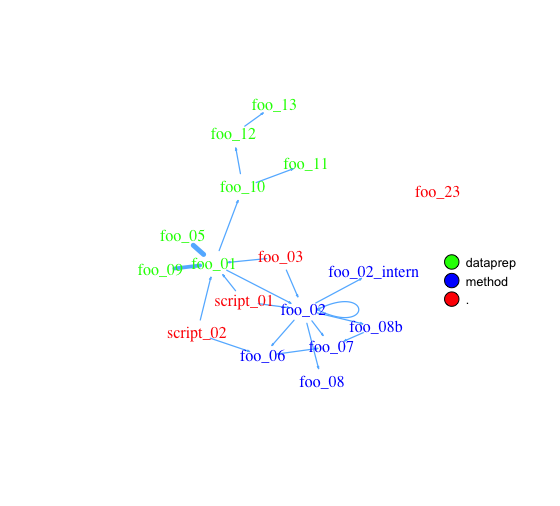Day 05 – little helper get_network

Jakob Gepp Blog

We at STATWORX work a lot with R and we often use the same little helper functions within our projects. These functions ease our daily work life by reducing repetitive code parts or by creating overviews of our projects. At first, there was no plan to make a package, but soon I realised, that it will be much easier to share and improve those functions, if they are within a package. Up till the 24th December I will present one function each day from `helfRlein`. So, on the 5th day of Christmas my true love gave to me…What can it do?

The aim of this little helper is to visualise the connections between R functions within a project as a flowchart. Herefore, the input is a directory path to the function or a list with the functions and the outputs are an adjacency matrix and an `igraph` object. As an example we use this folder with some toy functions:

``````net <- get_network(dir = "flowchart/R_network_functions/", simplify = FALSE)
g1 <- net\$igraph
``````

Input

There are five parameters to interact with the function:

• a path `dir` which shall be searched.
• a character vector `variations` with the function's definition string. The default is `c(" <- function", "<- function", "<-function")`.
• a `pattern` a string with the file suffix – the default is `"\\.R\$"`.
• a boolean `simplify` that removes functions with no connections from the plot.
• a named list `all_scripts` which is an alternative to `dir`. This is mainly just used for testing purposes.

For a normal usage it should be enough to provide a path to the project folder.

Output

The given plot shows the connections of each function (arrows) and also the relative size of the function's code (size of the points). As mentioned above, the output consists of an adjacency matrix and an `igraph` object. The matrix contains the number of calls for each function. The `igraph` object has the following properties:

• The names of the functions are used as label.
• The numer of lines of each function (without comments and empty one) are saved as the size.
• The folder's name of the first folder in the directory.
• A color corresponding to the folder.

With these properties you can improve the network plot for example like this:Code for example plot

``````library(igraph)

# create plots ------------------------------------------------------------
l <- layout_with_fr(g1)
colrs <- rainbow(length(unique(V(g1)\$color)))

plot(g1,
edge.arrow.size=.1,
edge.width = 5*E(g1)\$weight/max(E(g1)\$weight),
vertex.shape="none",
vertex.label.color=colrs[V(g1)\$color],
vertex.label.color="black",
vertex.size = 20,
vertex.color = colrs[V(g1)\$color],
edge.color="steelblue1",
layout = l)
legend(x=0,
unique(V(g1)\$folder), pch=21,
pt.bg= colrs[unique(V(g1)\$color)],
pt.cex=2, cex=.8, bty="n", ncol=1)
``````

Overview

To see all the other functions you can either check out our GitHub or you can read about them here.